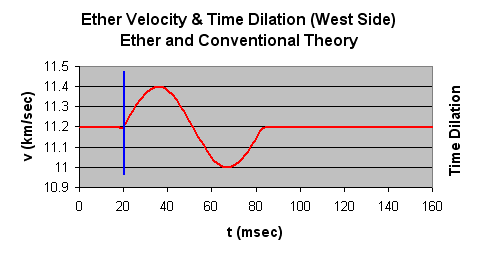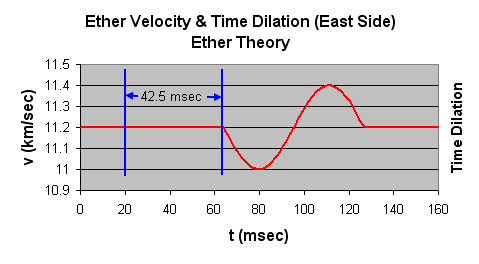top of page

"Do not follow where the path may lead. Go instead where there is no path and leave a trail."

- Muriel Strode -

## Gravitational Wave Detector

Ether Inflow

Figure 36 shows a sample graph of the effects that a gravitational wave could have on ether inflow. Normally, the speed of ether is a steady 11.2 km/sec at the earth's surface. A gravitational wave may momentarily cause the speed to increase to 11.4 km/sec, and then to decease to 11.0 km/sec. This will cause the laser's frequency to decrease and increase respectively. Figure 36 shows a sample gravitational wave (colored in red) coming in from the west side of the earth (see Figure 34).Figure 36

Since gravitational waves travel at the speed of light, 42.5 msec of time will elapse before the wave reaches the opposite side of the earth. Figure 37 illustrates this. Notice that the wave is inverted. This inversion is a result of the ether on the east side of earth flowing in an opposite direction to ether on the west side. Referring back to Figure 34, you can see that the ether is flowing from left to right on the west side of the earth, and from right to left on the east side. If the gravitational wave increases the ether's velocity on the west side of the earth, it will decrease ether velocity on the east side.

Ether theory predicts time dilation as illustrated in Figure 37 (with inversion). Conventional theory would predict time dilation as illustrated in Figure 38 (no inversion).Figure 37Figure 38

bottom of page